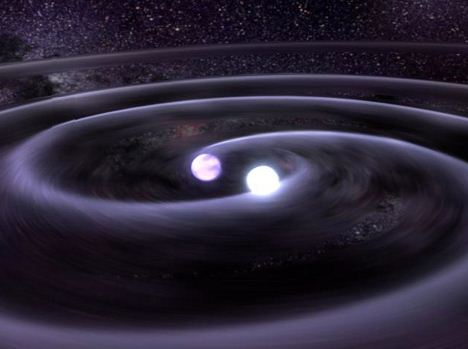Classical Mechanics

2D Dynamics WarmupThe above diagram shows a flying chair ride, where the central column rotates. Why are the people sitting in the seats thrown outwards?A kid is swinging on a rickety swing, where the attached ropes help to support her weight. If the ropes were to break, in which position would the swing most likely be?

In a circus, the joker wishes to show a daredevil act. In a big cylindrical drum, he presses himself against a vertical wall. Then the drum is rotated at a constant angular velocity $\omega$. Then suddenly the platform under his feet is removed. The joker remains stuck with the wall. There is no support to hold and he does not use any kind of glue.

If the mass of joker is $\SI{70}{\kilo\gram}$ and radius of the drum is $\SI{5}{\meter}$ and the coefficient of static friction between the drum and joker is 0.5 then, find the minimum angular velocity so that the joker does not fall on removing the platform.

Details: $g = \SI[per-mode=symbol]{10}{\meter\per\second\squared}.$A binary star is a system of two stars which revolve around their common center of mass.

In a binary star with two equally massive stars (of mass $m$ ), separated by a distance $r$, what will be the angular speed of revolution?

Note: Since the masses of the stars are equal, the center of mass lies exactly in the middle of the two stars, at distance $\frac{r}{2}$ from each point.
$G$ is the universal gravitational constant.Earth rotates about its axis, resulting in day and night. Because of this, all people and objects on Earth also move with it. If Earth's revolution around the Sun is neglected, and all people are at rest with respect to Earth then, what physical quantity is the same for all people on Earth?

×